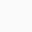# OVERVIEW

• A 45% (w/w) concentrated Potassium hydroxide solution is a clear colorless liquid that can be purchased from several commercial suppliers.
• % refers to solution concentration in percentage and “(w/w)” refers to solute and solvent amount given in grams (i.e., percentage by weight). This means a 45% (w/w) Potassium hydroxide solution contains 45 g of KOH per 100 g of Potassium hydroxide solution.
• The density of 45% (w/w) Potassium hydroxide solution is 1.456 g/ml at 25°C which means that the weight of the 1 ml of Potassium hydroxide solution is 1.456 g at 25°C.
• Molarity refers to the number of moles of the solute present in 1 liter of solution. In simple words, 1 mole is equal to the atomic weight of the substance. For example, 1 mole of KOH is equal to 56.11 g of KOH (molecular weight = 56.11).Calculation procedure:

Step 1: Calculate the volume of 100 g of Potassium Hydroxide.
Formula:

Density =  weight / volume or
Volume =  weight / density or
Volume of 100 g of Potassium Hydroxide : 100/1.456 =  68.6813 ml

Note: 45% (w/w) Potassium Hydroxide means that 100 g of Potassium Hydroxide solution contains 45 g of KOH.

The volume of 100 g of Potassium Hydroxide is 68.6813 ml. This means 45 g of KOH is present in 68.6813 ml of Potassium Hydroxide.

Step 2: Calculate how many grams of Potassium hydroxide is present in 1000 ml of Potassium Hydroxide solution.

68.6813 ml of Potassium Hydroxide contain   = 45 grams of KOH

1 ml of Potassium Hydroxide will contain       = 45/68.6813 grams of KOH

1000 ml of Potassium Hydroxide will contain = 1000 x 45/68.6813 = 655.2 grams of KOH

1000 ml of Potassium Hydroxide will contain 655.2 grams of KOH

Step 3: Calculate the number of moles of Potassium Hydroxide present in 655.2 g of Potassium Hydroxide solution.

56.11 grams of KOH is equal to 1 mole.

1 gram of KOH will be equal to 1/56.11 moles.

655.2 grams will be equal to = 655.2 x 1/56.11 = 11.677 moles

Therefore, we can say that 1 liter of Potassium hydroxide solution contains 11.677 moles or in other words molarity of 45% (w/w) Potassium Hydroxide is equal to 11.677 M.

Calculator – Calculate the molarity of concentrated Potassium Hydroxide (KOH)

Use Calculator to calculate the molarity of concentrated Potassium Hydroxide (KOH) when concentration is given in % by mass (w/w)

Molecular weight of Potassium Hydroxide (KOH) : 56.11 g/mol

Concentration of Potassium Hydroxide: % (wt/wt)
(Change the % (wt/wt) concentration)

Density of glacial Potassium Hydroxide: g/ml
(Change the density)

Molarity of Potassium Hydroxide: 11.677 M1.mark rico says:
1.admin says: